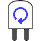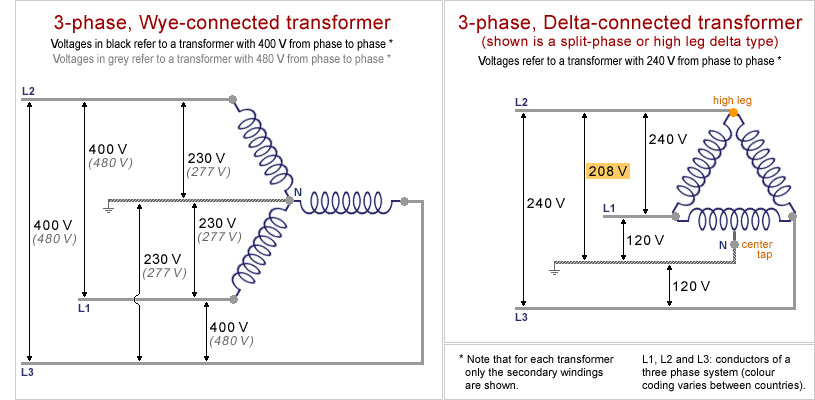3-Phase Transformers source of 120, 208, 230 and 277 Voltinfo pagedomestic NEMAheavy duty

 High voltage, three-phase alternating current is the common method to transport electric power over long distances. For domestic purposes it has to be transformed to the standard mains voltage, for example single phase 120 V in North America or single phase 230 V in Europe. The final step in the conversion process takes place in the distribution board of homes, commercial buildings etc. Two types of configurations can be used for the conversion of 3-phase, 400-480 V power to a single phase "household" voltage. The schemes of these Wye- or Delta-connected transformers are shown below.Wye-connected transformer (see left image above)*. In a wye system the voltage between any two phase conductors (L1, L2, L3) will always be the same in a three phase system. The voltage between any one of the phase conductors and neutral will be less than between two power conductors, i.e. the phase to phase voltage divided by the square root of 3. For example: if L1-L2, L2-L3, or L3-L1 = 400 Volt, then L1-N, L2-N or L3-N = 400/1.732 = 230 Volt (single phase). * alternative names are star-, or Y-connected transformer. The name Wye is related to the pronounciation of the letter Y.
 Delta-connected transformer. The voltage is the same between any two phases (L1-L2, L2-L3, or L3-L1). A special type of delta transformer has an additional neutral that is used to center-tap one of the windings (see right image above). In the example shown the winding connecting L1 and L3 is center-tapped and connected to neutral. The voltage between L1 and N, and L3 and N is divided into halves (120 Volt, single phase), while there is 208 Volt (120 x 1.732) between the high leg (L2) and neutral. Split-phase or high leg delta transformers are sometimes found in (older) three-phase electric power installations. In North America, the high leg delta system allows single-phase 120 V loads and 240 V three-phase loads both to be served by the same three-phase, four-wire distribution system. Notes: 1. The schemes above only show the secondary windings of a transformer. A full transformer consists of primary and secondary windings, which can be either of the same or different types: Y-Y, Y-Δ, Δ-Y or Δ-Δ (Δ = delta, Y = Wye), depending on the characteristics of the electric supply system and the demands given by the (household) loads. The image at right shows a Delta (primary winding) - Wye (secondary winding) scheme, the most commonly used 3-phase transformer type. 2. In older (European) 3-phase transformers, conductors L1, L2 and L3 might be designated as respectively R, S and T.D i g i t a l   M u s e u m   o fP l u g s   a n d   S o c k e t s